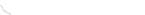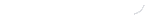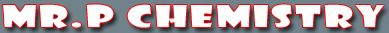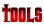activity seriesbond energybond lengthscalculatorcommon namesconversion factors: energyconversion factors: lengthconversion factors: massconversion factors: pressureconversion factors: temp.conversion factors: volumecovalent prefixesdensity solverdiatomic elementse-config. chartelement listgas law formulasKa's of polyprotic acidsKa's of weak acidsKb's of weak basesmetric conversion chartmole conversion chartmolecular geometriesorganic prefixesperiodic tableperiodic table (flash)pH/pOH converterpolyatomic ionspressure converterSI unitssolubility chartsolubility of salts rulessolubility product constantsstoichiometry charttemp. conversiontemp. formulasthermodynamic datavapor pressure of water
Density
 Density (d) is the ratio of the mass (m) of a substance to the volume (v) occupied by the substance. Pure water is used as the standard in measuring density. The density of pure water is 1.0 g/mL. If a substance has a density less than water, it will float; if a substance has a density greater than water, it will sink. How can you explain the soda cans in the photo below? Some float in water, others sink, all are unopened cans of soda.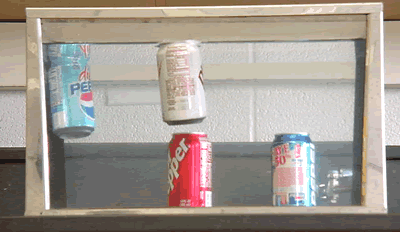Mass is expressed in grams (g). Volume of liquids is expressed in either milliliters (mL) or cubic centimeters (cc or cm3). Due to thieir low density, the volume of gasses is expressed in liters (L). Thus, density can be expressed as g/mL, g/cm3 or g/L (for gasses). The formula for density is: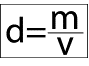You may also find these related tutorials helpfulCompounds in Crust of EarthDensityElements in Crust of EarthHow To Round NumbersMetric ConversionScientific NotationSI UnitsSignificant Figure CalculationsSignificant Figures